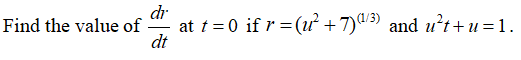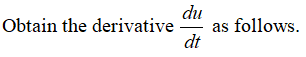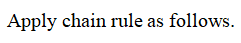# Find the value of dr/dt at t = 0 if r = (u2 + 7)^(1/3) and u^2t + u = 1.

Question
2 views

Find the value of dr/dt at t = 0 if r = (u2 + 7)^(1/3) and u^2t + u = 1.

check_circle

Step 1Step 2Step 3...

### Want to see the full answer?

See Solution

#### Want to see this answer and more?

Solutions are written by subject experts who are available 24/7. Questions are typically answered within 1 hour.*

See Solution
*Response times may vary by subject and question.
Tagged in
MathCalculus

### Derivative